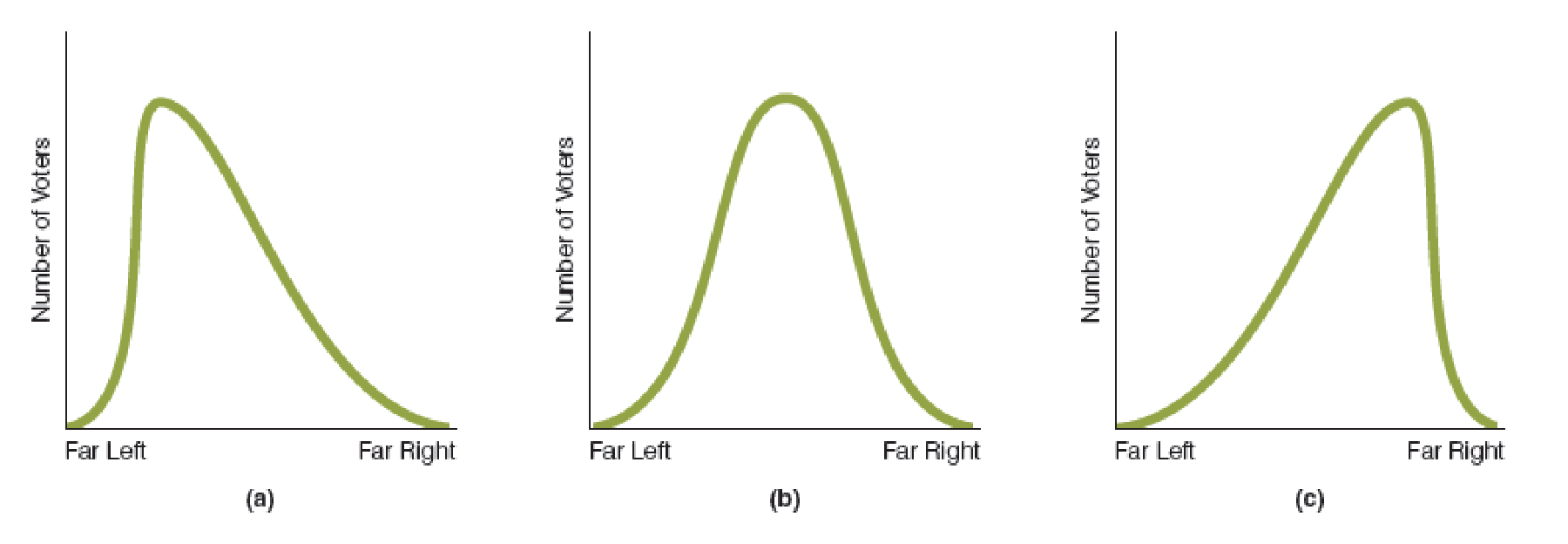Chapter 31, Problem 3WNG

Chapter
Section
Textbook Problem

In part (a) of the accompanying figure, the distribution of voters is skewed to the left; in part (b), the distribution is skewed neither left nor right; and in part (c), it is skewed right. Assuming a two-person race for each distribution, will the candidate who wins the election in (a) hold different positions from the candidates who win the elections in (b) and (c)? Explain your answer.To determine

Probability of winning in election.

Explanation

The figure shows the distribution of voters.

In Figure (1), the horizontal axis indicates the two spectra and the vertical axis represents number of voters. In Panel (a), the distribution of voters is skewed leftward. In Panel (b), it is neither skewed to both sides and in Panel (c), the distribution of voters skewed to the right...

Still sussing out bartleby?

Check out a sample textbook solution.

See a sample solution

The Solution to Your Study Problems

Bartleby provides explanations to thousands of textbook problems written by our experts, many with advanced degrees!

Get Started

Find more solutions based on key concepts